# GMAT Avengers Study Group: Triangles:

by on August 22nd, 2014

This Past Saturday, we did a session on ‘Triangles’ which show up all over the GMAT. We will often find them hiding in problems that seem to be about rectangles or other shapes.

To begin with, let’s share the links of all the articles that we shared on our Facebook Page.

## Types of Triangles

• Right Triangle - A right triangle is any triangle in which one of the angles is a right angle (90°).
• Isosceles Triangle - A triangle that has 2 equal angles and 2 equal sides (opposite the equal angles) is an isosceles triangle.
• Equilateral Triangle - A triangle that has 3 equal angles (all 60°) and 3 equal sides is an equilateral triangle.

## Important Properties of Triangles

• The sum of the three angles in a triangle is 180 degrees.
• Any triangle has three altitudes and three bases.
• If all the three angles in a triangle are equal, then all the three angles  equal  60 degrees.
• In a right triangle, the sum of two acute angles is 90 degrees.
• The sum of any two sides of a triangle must be greater than the third side.
• Sides correspond to their opposite angles. That is, the longest side is opposite the largest angle, and the smallest side is opposite the smallest angle.
• If two sides are equal, then the opposite angles are equal.
• If the two angles are equal, the opposite sides are equal.

## Pythagorean Theorem

This theorem applies only to Right Triangles. According to the theorem, the lengths of the 3 sides of a right triangle are related by the equation, where a and b are the lengths of the sides touching the right angle, also known as legs, and c is the length of the side opposite the right angle, also known as the hypotenuse.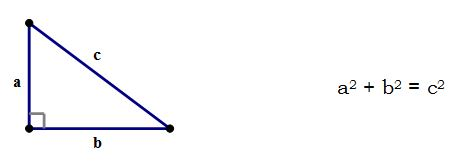We can always find the length of the third side of a right triangle if we know the lengths of the other two sides.

## Pythagorean Triplets to Remember for the GMAT

A few of the Pythagorean triplets are especially common and should be memorized. For each triplet, the first two numbers are the lengths of the sides that touch the right angle, and the third (and largest) number is the length of the hypotenuse. Remembering these will save us time on test day.

1. 3-4-5
2. 5-12-13
3. 8-15-17
4. 9-12-15
5. 12-16-20
6. 6-8-10 (this is 3-4-5 triplet doubled)
7. 10-24-26 (this is 5-12-13 doubled)

IMPORTANT : We can double, triple, or otherwise apply a common multiplier to these lengths. 3-4-5 should be thought of as a ratio of 3:4:5.

## GMAT’s Favorite Triangles

The two special triangles are right triangles with special angles and side. Like all right triangles, they satisfy the Pythagorean Theorem. These two triangles are “special” because, with just a couple pieces of information, we can figure out all their properties.

1) 45-45-90 Triangle. It is also known by the following names:

• The Isosceles Right Triangle
• The 1-1-Triangle

Here, the triangle is an isosceles triangle, with the sides in the ratio 1:1:. We can multiply the sides by any common number we like to scale this up.

2) 30-60-90 Triangle. It is also known by the following names:

• The Half Equilateral Triangle
• The 1--2 Triangle

Here, the triangle is an equilateral triangle, with the sides in the ratio 1::2. We can multiply the sides by any common number we like to scale this up.

## Some Important Formulae

• The perimeter of a triangle is the sum of the lengths of all 3 sides.
• The area of a triangle is. (The perimeter of a triangle is the sum of the lengths of all 3 sides. Remember that any side of a Triangle can act as a base and the height is the altitude of the triangle which is nothing but a line drawn perpendicular from a vertex to an opposite base.)
• The area of an equilateral triangle is. (The whole expression is divided by 4)

## Geometrically Similarity

When two geometric figures are the same shape and same size, they are congruent. every single piece of one figure is absolutely identical to the corresponding piece of the other figure. They are identical copies of one another.

When two geometric figures are the same shape and different sizes, they are similar. The larger one is a bigger copy of the smaller one. Each is a “scaled up” or “scaled down” version of the other. All the lengths will be different, but all the angles will be the same.

It is important to note that the lengths of similar figures are proportional.

## Problem Solving Practice

1)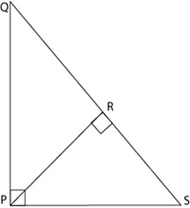In the figure above, if the length of PQ is 4 and the length of PS is 3, what is the length of line PR?

A) 9/4

B) 12/5

C) 16/5

D) 15/4

E)  20/3

2)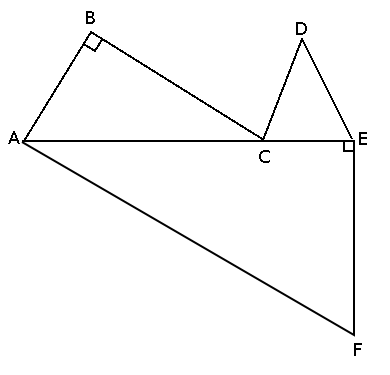In the figure above, triangle CDE is an equilateral triangle with side length 3, AF = 10, EF = 6, and AB = DE. What is the perimeter of the figure?

A) 45

B) 36

C) 29

D) 24

E) 16

3) A regular octagon (a polygon with 8 sides of identical length and 8 identical interior angles) is constructed. Next, an equilateral triangle (with sides identical in length to those of the octagon) is attached to each side of the octagon, such that each side of the octagon coincides exactly with the side of the triangle. Finally, each triangle is folded over that coincident side onto the octagon, covering part of the latter’s area. Approximately what proportion of the area of the octagon is left uncovered?

A) 60%

B) 50%

C) 40%

D) 30%

E) 20%

4) A total of m different points are selected on a particular line, and a total of n different points are selected on another line parallel to the first, where each of m and n is greater than 1. In how many different ways can a triangle be made with its vertices at three of the selected points?

A)B)C)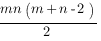D)E)5)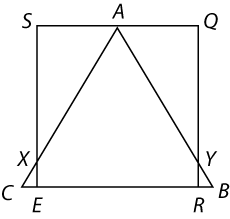In the diagram above, triangle ABC is equilateral, figure SQRE is a square, and A is the midpoint of SQ. If the perimeter of triangle ABC is 6 inches, what is the length, in inches, of segment RY?

A) 0.5

B) 1.5

C)D)E)6)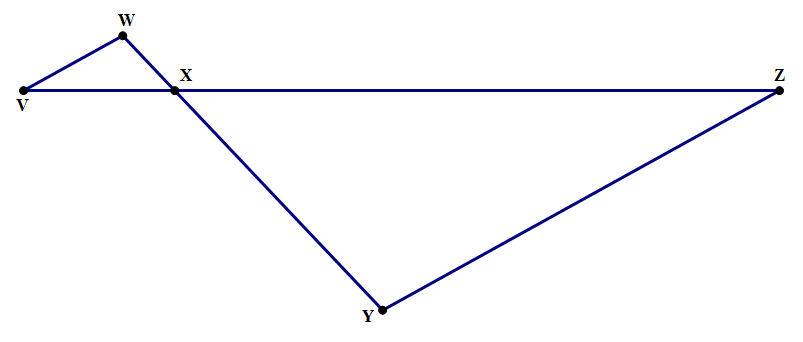In the diagram above, ∠W = ∠Y and VX is one fifth of VZ. If the area of triangle VWX is 5, what is the area of triangle XYZ?

1) 25

2) 40

3) 50

4) 80

5) 125

## Data Sufficiency Practice

1)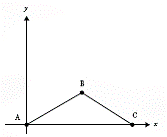In the rectangular coordinate system shown above, what is the area of triangle ABC?

(1) The coordinates of B are (3,4).

(2) The coordinates of C are (6,0)

2) What is the perimeter of isosceles triangle LMN?

(1) Side LM has a length of 4

(2) Side MN has a length of3)  In isosceles triangle DEF, what is the measure of angle E?

(1) Angle D measures 42 degrees

(2) Angle F measures 96 degrees

4)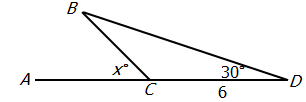If CD = 6, what is the length of BC in the figure above ?

(1) BD =(2) x = 60

5)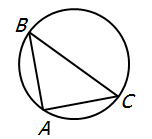If the circle shown above has radius 6, what is the area of the triangle?

(1) AC = AB

(2) BC = 12

6)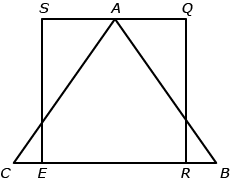In the figure above, SQRE is a square, AB = AC, and AS = AQ. What is the difference between the perimeter of triangle ABC and the perimeter of square SQRE?

(1) The length of RE is 4 times the length of BR.

(2) The area of triangle ABC is 75% of the area of square SQRE.

Problem Solving

1. B
2. C
3. D
4. C
5. C
6. D

Data Sufficiency

1. C - Both statements 1 and 2 together are sufficient to answer the question but neither statement is sufficient alone
2. E - Statements (1) and (2) TOGETHER are NOT sufficient and more information is needed to answer the question
3. B - Statement 2 alone is sufficient but statement 2 alone is not sufficient to answer the question asked
4. D - Each statement alone is sufficient to answer the question
5. C - Both statements 1 and 2 together are sufficient to answer the question but neither statement is sufficient alone
6. D - Each statement alone is sufficient to answer the question

Make sure you check out the event Facebook page to see all the comments that were being exchanged during the live session.

***

RSVP NOW if you want to join me and fellow GMAT Avengers take on SC Subject-Verb Agreement questions.

••